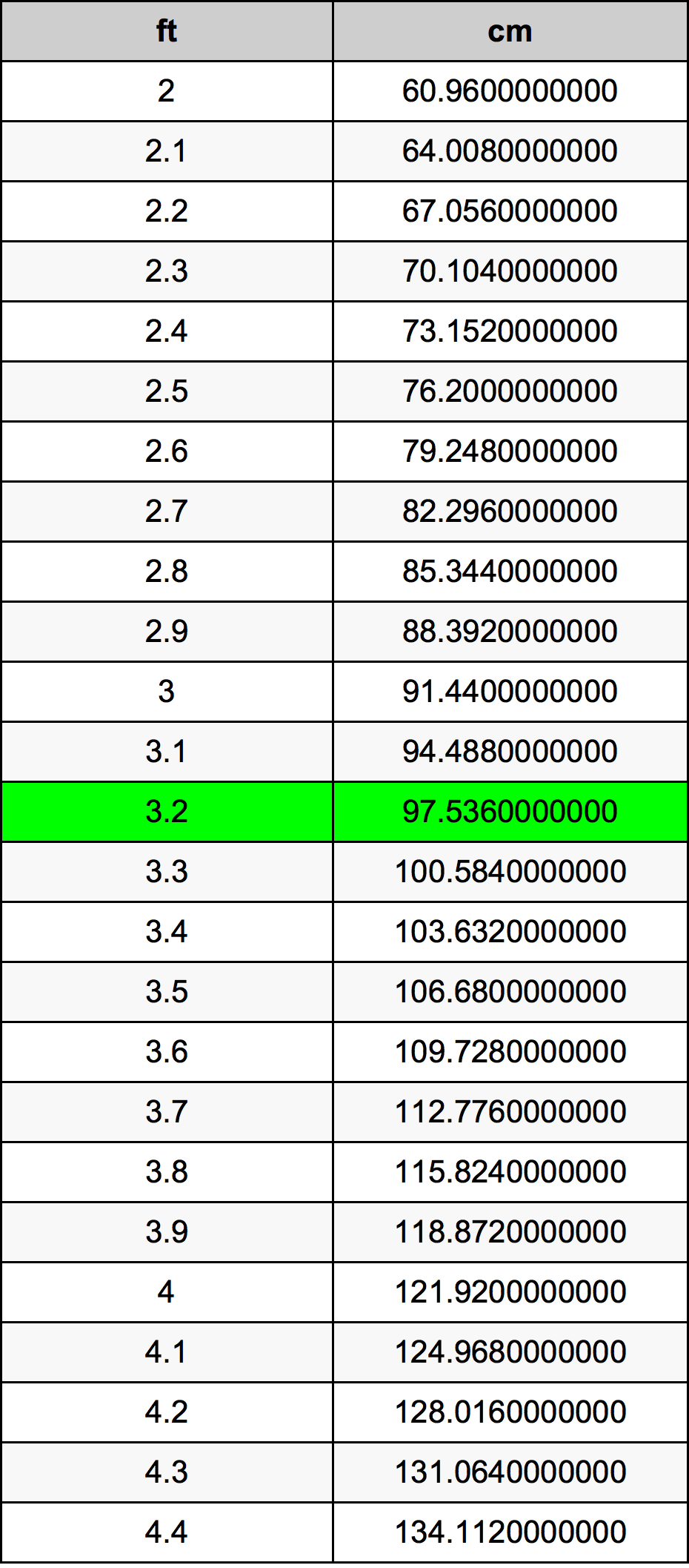Feet To Cm

# 3.2 ft to cm3.2 Feet to Centimeters

ft
=
cm

## How to convert 3.2 feet to centimeters?

 3.2 ft * 30.48 cm = 97.536 cm 1 ft
A common question is How many foot in 3.2 centimeter? And the answer is 0.1049868766 ft in 3.2 cm. Likewise the question how many centimeter in 3.2 foot has the answer of 97.536 cm in 3.2 ft.

## How much are 3.2 feet in centimeters?

3.2 feet equal 97.536 centimeters (3.2ft = 97.536cm). Converting 3.2 ft to cm is easy. Simply use our calculator above, or apply the formula to change the length 3.2 ft to cm.

## Convert 3.2 ft to common lengths

UnitLengths
Nanometer975360000.0 nm
Micrometer975360.0 µm
Millimeter975.36 mm
Centimeter97.536 cm
Inch38.4 in
Foot3.2 ft
Yard1.0666666667 yd
Meter0.97536 m
Kilometer0.00097536 km
Mile0.0006060606 mi
Nautical mile0.0005266523 nmi

## What is 3.2 feet in cm?

To convert 3.2 ft to cm multiply the length in feet by 30.48. The 3.2 ft in cm formula is [cm] = 3.2 * 30.48. Thus, for 3.2 feet in centimeter we get 97.536 cm.

## 3.2 Foot Conversion Table## Alternative spelling

3.2 ft to Centimeters, 3.2 ft in Centimeters, 3.2 Feet to Centimeter, 3.2 Feet in Centimeter, 3.2 Foot to Centimeters, 3.2 Foot in Centimeters, 3.2 ft to cm, 3.2 ft in cm, 3.2 Feet to cm, 3.2 Feet in cm, 3.2 Foot to Centimeter, 3.2 Foot in Centimeter, 3.2 ft to Centimeter, 3.2 ft in Centimeter